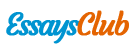# Eecs 2210 - Electronic Circuits and Devices

Autor:   •  February 24, 2018  •  956 Words (4 Pages)  •  196 Views

Page 1 of 4

...

Values of current and voltage across the diode at various source voltages are shown in the following table.

Table 3: Measured values of Id for varying Vd

Source Voltage (V)

Vd (across diode) (V)

Id (diode current) (mA)

0.10

0.097

0.001

0.25

0.247

0.050

0.5

0.489

0.070

0.6

0.563

0.330

0.7

0.610

0.860

0.8

0.636

1.550

0.9

0.655

2.320

1.0

0.670

3.136

1.2

0.690

4.830

1.3

0.701

5.710

1.5

0.715

7.480

3.0

0.775

21.320

The graph on the next page summarizes the relationship between the voltage across, and the current through a given diode.

[pic 2]

Figure 2: i-v characteristics of the diode used in the lab (1N4148)

[pic 3]

Figure 2a : Same graph as in figure 2, produced using excel

PART 2:

The following figure shows the schematic used in the second part of the lab.

[pic 4]

Figure 3: Simplified Circuit diagram part 2.

We chose a resistor of 1kΩ as recommended in the lab. This allowed the current to reach a value of approximately 13mA before saturation.

The following picture shows a screenshot of the oscilloscope for this part.

[pic 5]

Figure 4: i-v curve of the diode on an oscilloscope

PART 3:

Following diagram shows the circuit diagram for part 3.

[pic 6]

Figure 5: Part 3 schematic

Figure 6 and 7 show the output of the rectifier with and without capacitor shunting respectively

[pic 7]

Figure 6: Rectified output from bridge rectifier

[pic 8]

Figure 7: Filtered output after adding the shunt capacitor

PART 4:Following is a picture from the diode data sheet which can be used to find the exact value of the differential diode voltage at a current.

[pic 9]

https://www.fairchildsemi.com/datasheets/1N/1N914.pdf

The calculation of diode resistance was done manually by finding the slope at 10 mA (as instructed by the TA), The slope was found to be 4.4 ohms (44mV / 10 mA )

[pic 10]

DISCUSSION

Part 1:

The i-v characteristics measured using a DMM are a very good approximation of the theoretical i-v characteristic’s for a diode.

Part 2:

What limits the ability to measure the i-v curve to higher voltages/currents in this setup?

The diode is connected to an amplifier and an amplifier has a certain saturation current and voltage determined by its power sources. We used a resistor of 1 kilo Ohms at which the current saturated at about 13mA(output), at a lower resistance the saturation would have occurred at a lower current output. Since low resistance means more current in the feedback loop.

Part 4:

The value of dynamic resistance of the diode at about 10mA was more or less the same using the DMM method or the oscilloscope method. The value given in the datasheet is 5 ohms, however based on my calculation the value obtained in part 4 was 4.4 Ohms.

The difference is about 12 percent (.6 / 5 * 100% ) which can be attributed to the experimental errors and the inaccuracy of calculating slope using a manual method.

CONCLUSION

There can be 4 major conclusions drawn from this lab

- The behavior

...